ISEE Middle Level Math : How to multiply fractions

Example Questions

1 2 3 4 5 7 Next →

Example Question #61 : How To Multiply Fractions

Solve the following: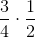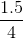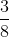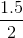Explanation:

To multiply equations, we will multiply the numerators together, then we will multiply the denominators together.  So, we get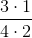Example Question #62 : How To Multiply Fractions

Multiply the following: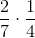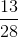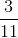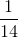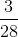Explanation:

To multiply fractions, we will multiply the numerators together, then we will multiply the denominators together.  Note that we do NOT need to find a common denominator.

So, we will multiply: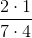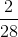Example Question #63 : How To Multiply Fractions

Multiply the following: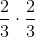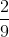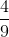Explanation:

To multiply fractions, we will multiply the numerators together, then we will multiply the denominators together.

So, we get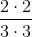1 2 3 4 5 7 Next →